# NCERT Solutions for Class 9 Maths Exercise 5.2## myCBSEguide App

CBSE, NCERT, JEE Main, NEET-UG, NDA, Exam Papers, Question Bank, NCERT Solutions, Exemplars, Revision Notes, Free Videos, MCQ Tests & more.

NCERT solutions for Class 9 Maths Introduction to Euclids Geometry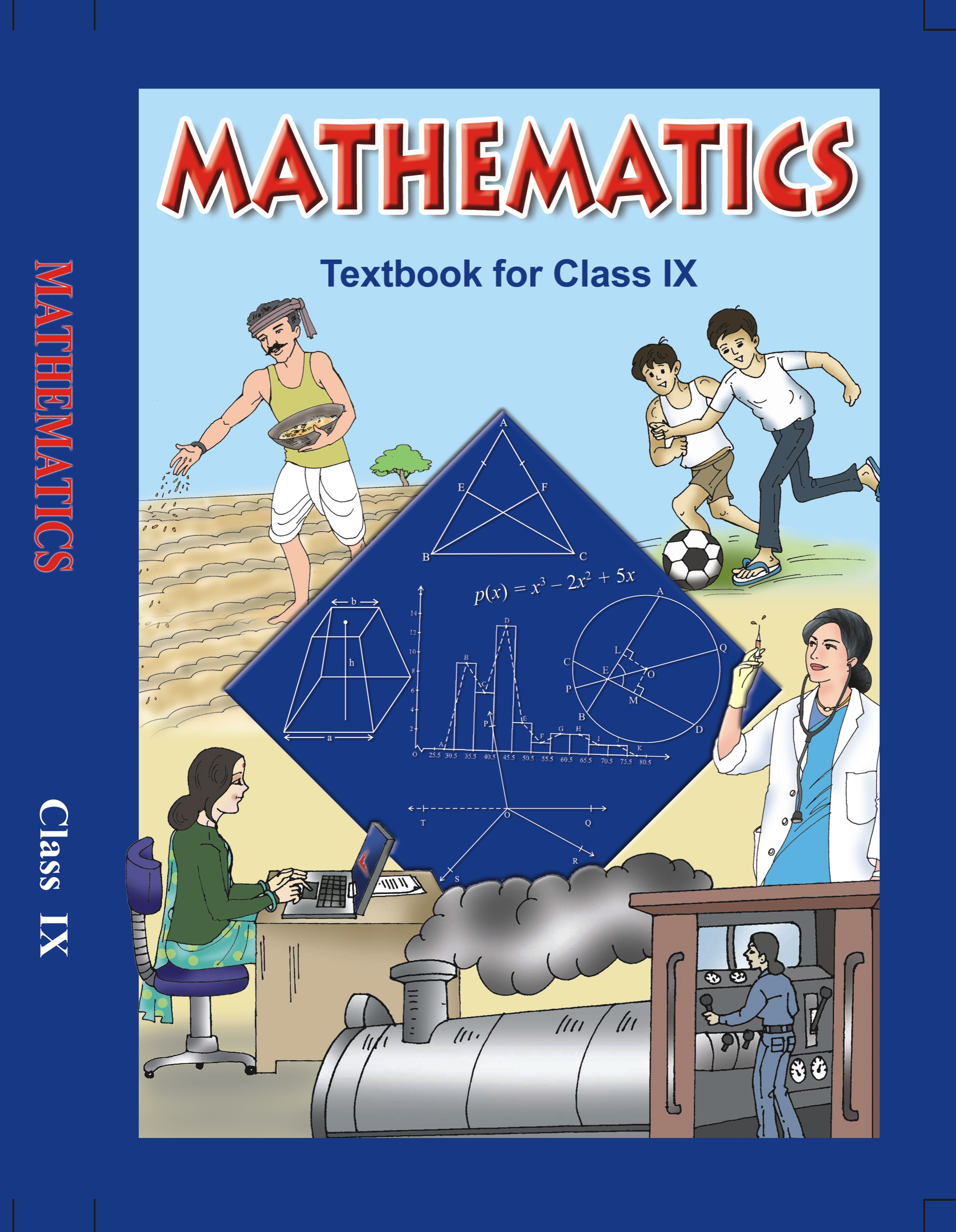## NCERT Solutions for Class 9 Mathematics Introduction to Euclids Geometry

###### 1. How would you rewrite Euclid’s fifth postulate so that it would be easier to understand?

Ans. We need to rewrite Euclid’s fifth postulate so that it is easier to understand.

We know that Euclid’s fifth postulate states that “No intersection of lines will take place when the sum of the measures of the interior angles on the same side of the falling line is exactly 180°.”

We know that Play fair’s axiom states that “For every line l and for every point P not lying on l, there exists a unique line m passing through P and parallel to l”.

The above mentioned Play fair’s axiom is easier to understand in comparison to the Euclid’s fifth postulate.

Let us consider a line l that passes through a point p and another line m. Let these lines be at a same plane.

Let us consider the perpendicular CD on l and FE on m.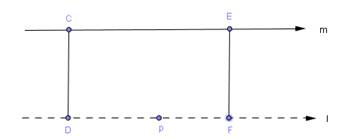From the above figure, we can conclude that CD = EF.

Therefore, we can conclude that the perpendicular distance between lines m and l will be constant throughout, and the lines m and l will never meet each other or in other words, we can say that the lines m and l are equidistant from each other.

NCERT Solutions for Class 9 Maths Exercise 5.2

###### 2. Does Euclid’s fifth postulate imply the existence of parallel lines? Explain.

Ans. We need to verify whether Euclid’s fifth postulate imply the existence of parallel lines or not.

The answer to the above statement is Yes.

Let us consider two lines m and l.

In the figure given below, we can conclude that the lines m and l will intersect further.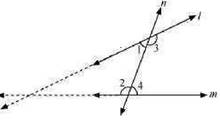From the figure, we can conclude that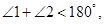andWe know that Euclid’s fifth postulate states that “No intersection of lines will take place when the sum of the measures of the interior angles on the same side of the falling line is exactly 180°.”

Let us consider lines l and m.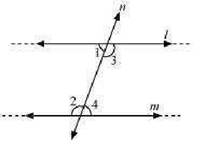From the above figure, we can conclude that lines l and m will never intersect from either side.

Therefore, we can conclude that the lines l and m are parallel.

## NCERT Solutions for Class 9 Maths Exercise 5.2

NCERT Solutions for Class 9 Maths PDF (Download) Free from myCBSEguide app and myCBSEguide website. Ncert solution class 9 Maths includes text book solutions from Mathematics Book. NCERT Solutions for CBSE Class 9 Maths have total 15 chapters. 9 Maths NCERT Solutions in PDF for free Download on our website. Ncert Maths class 9 solutions PDF and Maths ncert class 9 PDF solutions with latest modifications and as per the latest CBSE syllabus are only available in myCBSEguide.

## CBSE app for Class 9

To download NCERT Solutions for Class 9 Maths, Computer Science, Home Science,Hindi ,English, Social Science do check myCBSEguide app or website. myCBSEguide provides sample papers with solution, test papers for chapter-wise practice, NCERT solutions, NCERT Exemplar solutions, quick revision notes for ready reference, CBSE guess papers and CBSE important question papers. Sample Paper all are made available through the best app for CBSE students and myCBSEguide website.